Author: Oscar Cronquist Article last updated on August 10, 2020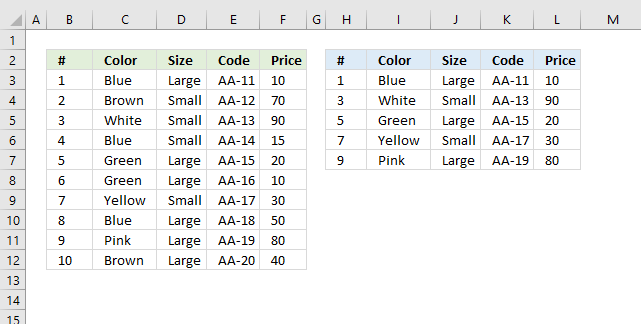This article demonstrates a formula and a VBA macro that returns every n-th row from a given cell range. The image above shows a list in cell range B2:F12 and a formula in cell range H3:L7 extracting every other row from cell range B3:F12.

The following link takes you to an article that explains how to copy rows matching a condition: Extract multiple records based on a condition

Formula in cell H3:

=INDEX(\$B\$3:\$F\$12, SEQUENCE(5, , , 2), SEQUENCE(, 5))

The SEQUENCE function was introduced in January 2020 and is available to Excel 365 subscribers. It generates a series of numbers based on the rows, columns, start and step arguments. The columns, start and step arguments are optional.

SEQUENCE(rows,[columns],[start],[step])

I will now describe another formula below for older Excel versions.

Formula in cell H3:

=INDEX(\$B\$3:\$F\$12,ROWS(\$A\$1:A1)*2-1,COLUMNS(\$A\$1:A1))

This formula is smaller, however, you need to copy cell H3 and paste to adjacent cells as far as needed both horizontally and vertically. This is not necessary with the first formula above as it expands automatically, this behavior by the formula is called spilling and is a feature for Excel 365 subscribers.

### Explaining formula for older versions in cell H3

The steps below describe how to get every other row, however, I will also explain below what to change in order to get every nth row.

#### Step 1 - INDEX function

The INDEX function lets you fetch a given value, it requires a row number and a column number in order to return a specific value from a given cell range.

The row and column arguments allow you to specify which value to get from the cell range.

INDEX(cell_ref, [row], [col])

#### Step 2 - Calculate row number

To get every nth row we need to create a formula that returns a number series based on a step value. The ROWS function returns a number which represents the number of rows a cell range contains.

The cell reference \$A\$1:A1 expands when you copy cell H3 and paste to cells below, the first part is an absolute cell reference \$A\$1 which means it is locked to cell A1.

The second part A1 is a relative cell reference. It changes when the cell is copied to cells below as far as needed.

ROWS(\$A\$1:A1)*2-1

becomes

1*2-1

and returns 1.

#### Step 3 - Calculate column number

The COLUMNs function returns the number of columns in a cell range. The arumnet contains a cell range that expands as well when the cell is copied to cells below.

COLUMNS(\$A\$1:A1))

returns 1.

#### Step 4 - Return value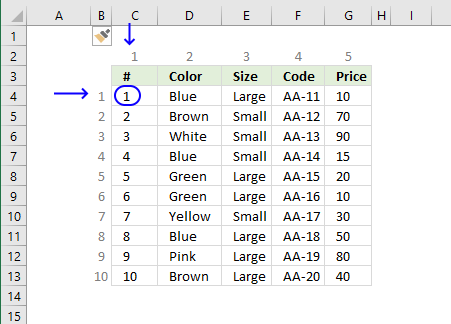INDEX(\$B\$3:\$F\$12,ROWS(\$A\$1:A1)*2-1,COLUMNS(\$A\$1:A1))

becomes

INDEX(\$B\$3:\$F\$12,1,1)

becomes

\$B\$3

and returns 1 in cell H3.

### What to change in formulas if I want every nth row?

Excel 365 formula:

=INDEX(\$B\$3:\$F\$12, SEQUENCE(5, , , 2), SEQUENCE(, COLUMNS(\$B\$3:\$F\$12)))

The first argument in the SEQUENCE function specifies the number of values the number series will contain. The last argument is the step value to use.

SEQUENCE(5, , , 2)

returns {1; 3; 5; 7; 9}

If you want every fifth row and a total of twenty rows then the SEQUENCE function becomes:

SEQUENCE(20, , , 5)

returns {1; 6; 11; 16; 21; 26; 31; 36; 41; 46; 51; 56; 61; 66; 71; 76; 81; 86; 91; 96}

SEQUENCE(20, ,2 , 5)

returns

{2; 7; 12; 17; 22; 27; 32; 37; 42; 47; 52; 57; 62; 67; 72; 77; 82; 87; 92; 97}

Formula for all Excel versions:

=INDEX(\$B\$3:\$F\$12,ROWS(\$A\$1:A1)*2-1,COLUMNS(\$A\$1:A1))

You want to change the bolded numbers in the above formula to get every nth value.

n-(n-1)

Example 1, to get every fourth number change 2-1 to 4-3.

Example 2, to fetch every 7th row change 2-1 to 7-6.

## Copy every nth row - VBA macro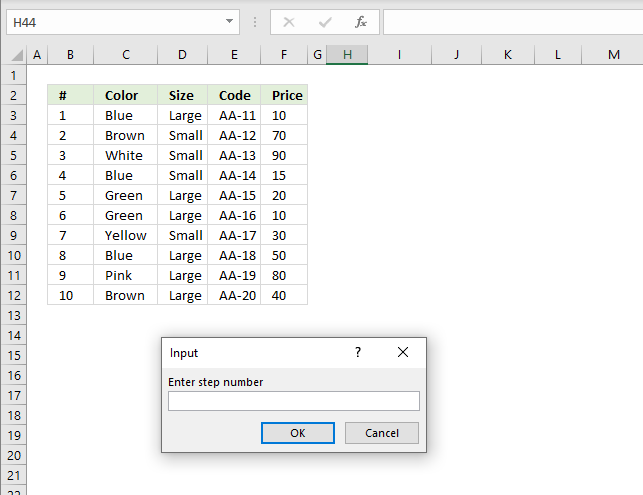The image above shows the macro displaying an inputbox asking the Excel user for a step value.

```Sub CopyNthRow()

sn = CInt(Application.InputBox("Enter step number", , , , , , , 1))

st = CInt(Application.InputBox("Enter starting row number", , , , , , , 1))

Set Rng = Application.InputBox(Prompt:="Select cell range:", _
Title:="Copy every nth rows", _

'Create new worksheet

'Save number 1 to variable r
r = 1

'Save number of rows in cell range
co = CInt(Rng.Rows.CountLarge)

'Copy rows based on step number
For i = st To CInt(co) Step sn

'Paste to new worksheet
Rng.Rows(i).Copy Ws.Range("A" & r)

r = r + 1

'Continue with next number based on variable i
Next i

End Sub

```

### Where to put the code?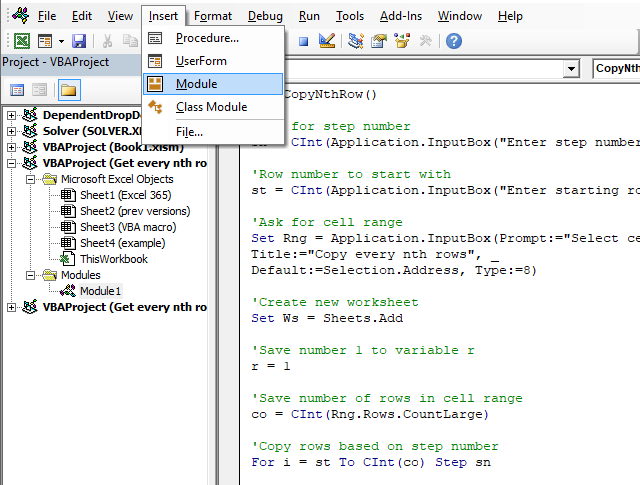1. Press Alt + F11 to open the Visual Basic Editor.
2. Press with left mouse button on "Insert" on the top menu, see image above.
3. Press with left mouse button on "Module". A module named Module1 appears in the Project Explorer, see image above.
4. Paste code to window.
Note, save your workbook with file extension *.xlsm (macroenabled workbook) to attach the code to your workbook.

### How to run macro?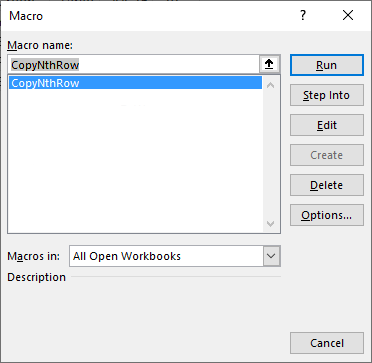1. Press Alt + F8 to open the Macro dialog box.
2. Press with mouse on the macro name, in this case CopyNthRow.
3. Press with left mouse button on "Run" button.

### What happens when you run the macro?The macro asks the user for a step number (n), a start row number, and a cell range to use. It will now copy every nth row from the supplied cell range to a new worksheet.

### Get the Excel fileGet-every-nth-row-from-list.xlsm